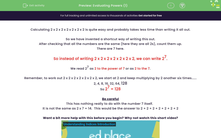# Evaluate Powers

In this worksheet, students will evaluate powers.Key stage:  KS 3

Curriculum topic:   Number

Curriculum subtopic:   Understand Integer Powers/Real Roots

Difficulty level:#### Worksheet Overview

Calculating 2 x 2 x 2 x 2 x 2 x 2 x 2 takes a long time to write out.

So we have invented a shortcut way of writing it.

After checking that all the numbers are the same (here they are all 2s), count them up.

There are 7 here.

So, instead of writing 2 x 2 x 2 x 2 x 2 x 2 x 2, we can write 27

We read 27 as 2 to the power of 7 or as 2 to the 7.

This is what 27 means:

2 x 2 = 4

4 x 2 = 8

8 x 2 = 16

16 x 2 = 42

32 x 2 = 64

64 x 2 = 128

So, 27 = 128

Be careful

This has nothing to do with the number 7 itself!

It is not the same as 2 x 7 = 14.  This would be the answer to 2 + 2 + 2 + 2 + 2 + 2 + 2

Let's get started on some questions!### What is EdPlace?

We're your National Curriculum aligned online education content provider helping each child succeed in English, maths and science from year 1 to GCSE. With an EdPlace account you’ll be able to track and measure progress, helping each child achieve their best. We build confidence and attainment by personalising each child’s learning at a level that suits them.

Get started